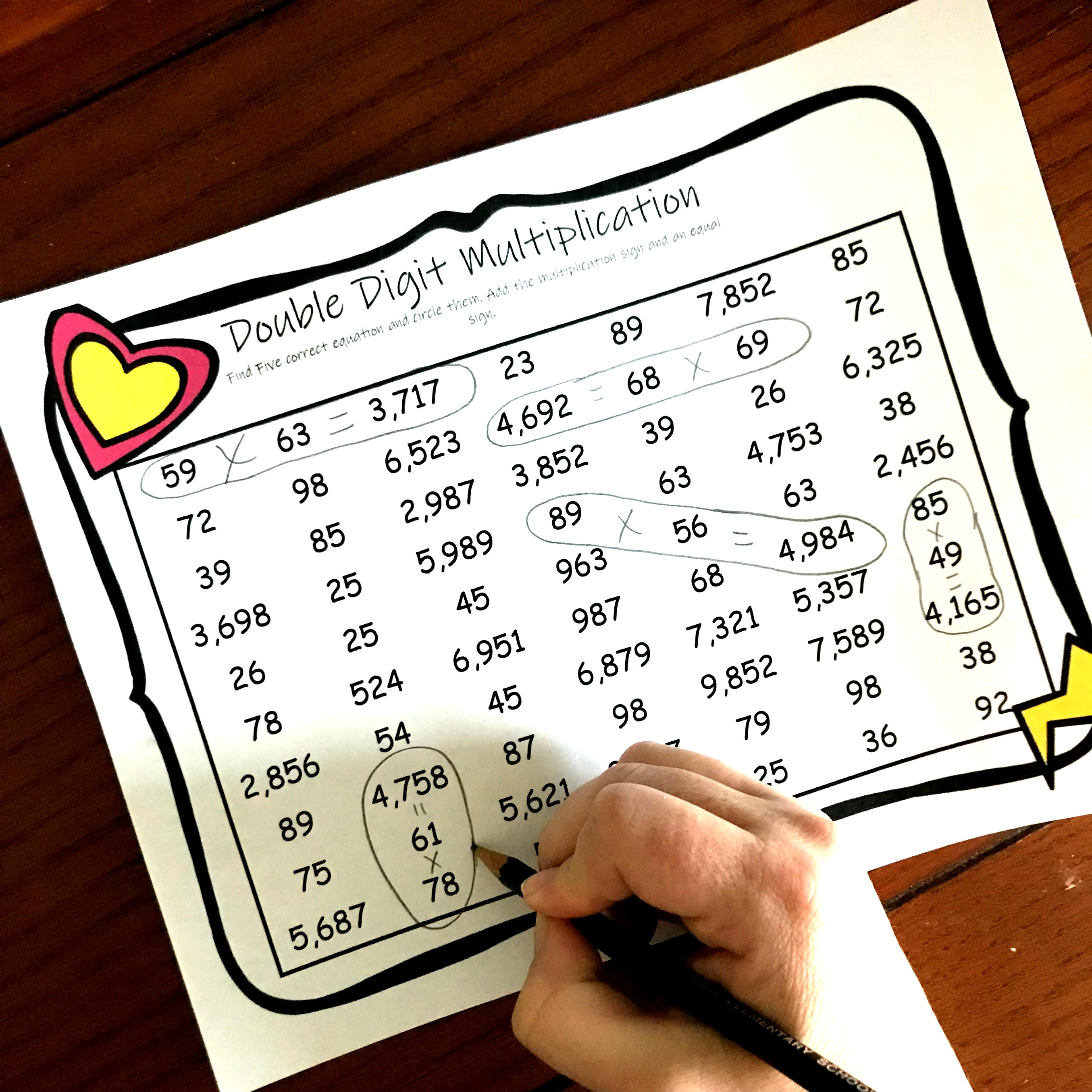# FREE Two Digit Multiplication Worksheets with a Fun Twist

These FREE Two Digit Multiplication Worksheets making practicing multiplication fun. The children search through numerous equations to find five correct ones. Also great for rounding practice.

Let’s face it. There are some skills that just have to be practiced over and over again for children to become successful at them.

Two digit multiplication is one of them. Though some children will pick it up quick others will need multiple exposures and numerous time to practice it.

So today, I have a two-digit multiplication worksheet that gets children practicing rounding and solving two-digit multiplication problems….but it is more fun than just a regular worksheet. It comes with a fun twist.

## Before the Worksheet

Obviously, before our kiddos attempt this page, they need a little training in this skill. I know we are all familiar in the traditional method, but I would like to encourage you to allow your children to explore different methods. What works for one child, may not work for another. Our goal is for the children to get the right answer, not do it a certain way.

I love exposing my class to numerous ways of doing a math problem and then letting the children choose which way works best for them.

Here are a few different methods, with step by step instructions on how to solve them.

Partial Products

Area Model

Using Arrays and Base Ten Blocks

Lattice Multiplication

## Prep-Work

Oh, you will love this! There really isn’t any!!!

1. First, print off the pages you will need. To save paper make sure you don’t print off the answer keys.
2. Next, grab some pencils and markers and you are ready to go.

You could also make this a center and only make a few copies. Put the pages you want them to complete in sheet protectors and provide dry erase markers. Then have the students write down the correct equations on a piece of paper for a grade or accountability.

## Two Digit Multiplication Worksheets: How to Use

These worksheets are just like word searches, except the children will be solving two-digit multiplication problems and circling equations that are correct.

It is important that we give our children strategies for finding the equations, or this may be a little overwhelming.

One strategy would be to have them estimate the answer before they solve.

When I look at the top of this page, the first two numbers I see are 59 and 63.

I begin by rounding to the nearest 10, which is 60 x 60 and that equals 3,600. This is pretty close to the 3,717 that is next to those numbers, so I will go ahead and multiply 59 x 63. And surprise, I get 3,717. I just found my first correct equation.

But on this page, my first two numbers are 15 and 35. Rounding to the nearest 10, I get 20 and 30. 20 x 30= 600. I know that I rounded up on both of those numbers so there is no way the answer is 765, so I’m going to move on and find another equation.

Even though there are only five correct equations on the sheet, the children will have solved numerous rounding problems and two digit multiplication problems by the time they finish.

I hope they enjoy this Two Digit Multiplication Worksheets with a Fun Twist!

You’ve Got This,

Rachel

You may also like:

Or get all my multiplication and division printables in one easy download.